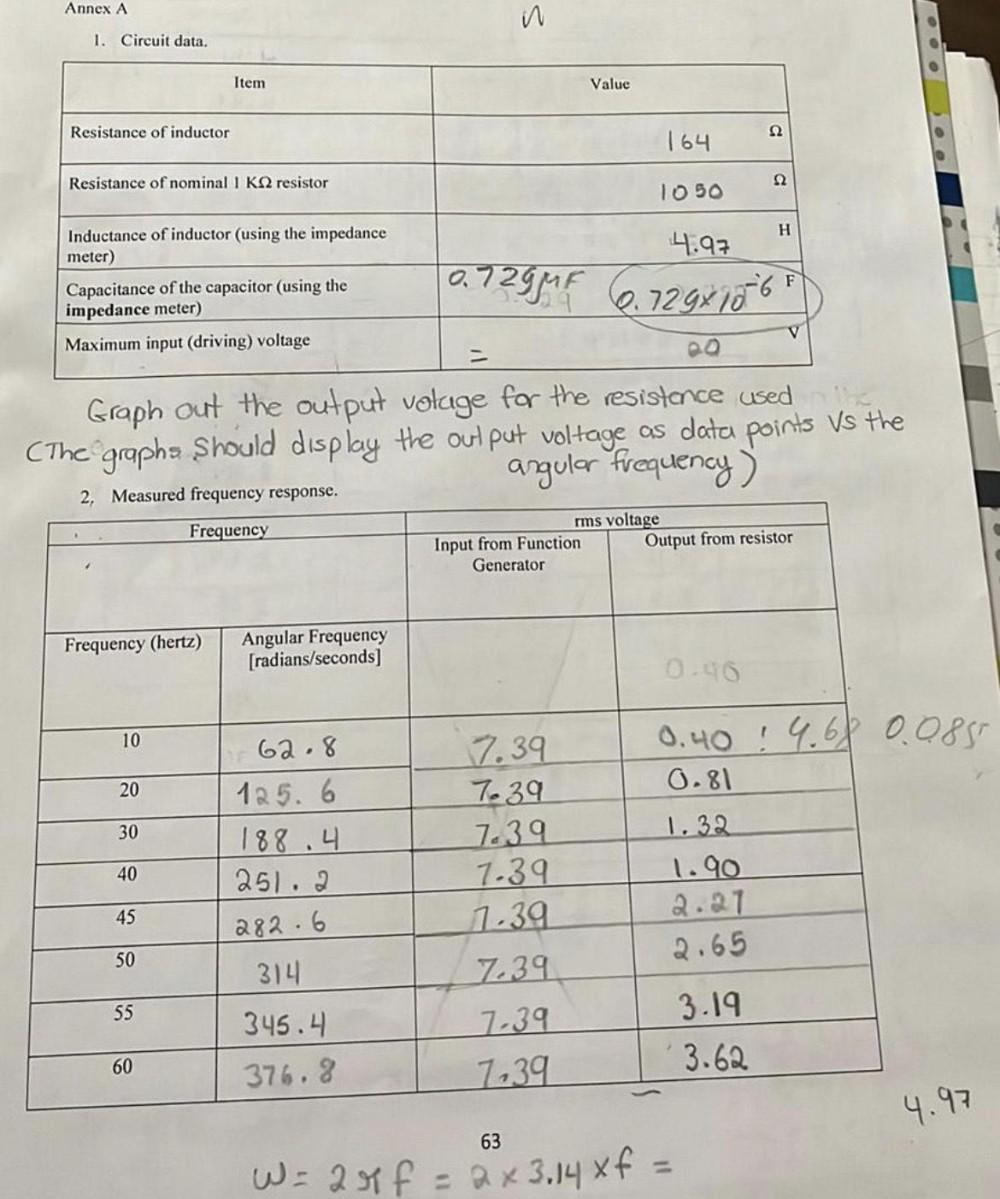Question:

# Annex A 3 Foo 1. Circuit data. Item Value Resistance of inductor 12 164 Resistance of nominal 1 K22 resistor 12 1050 H InductancAnnex A 3 Foo 1. Circuit data. Item Value Resistance of inductor 12 164 Resistance of nominal 1 K22 resistor 12 1050 H Inductance of inductor (using the impedance meter) 4.97 0.729ur 6.729x1806 Capacitance of the capacitor (using the impedance meter) Maximum input (driving) voltage V Graph out the output voluge for the resistance used The graphs should display the output voltage as data points Vs the angular frequency) 2. Measured frequency response. Frequency rms voltage Input from Function Output from resistor Generator Frequency (hertz) Angular Frequency (radians/seconds) 0.96 10 20 62.8 125.6 188.4 251.2 30 7.39 7.39 7.39 7.39 1.39 0.40 9.68 0.085 0.81 1.32 1.90 2.21 2.65 40 45 282.6 50 314 7.39 55 345.4 7.39 7,39 3.19 3.62 60 376.8 4.97 63 W = 20 f = ax 3,14 xf =